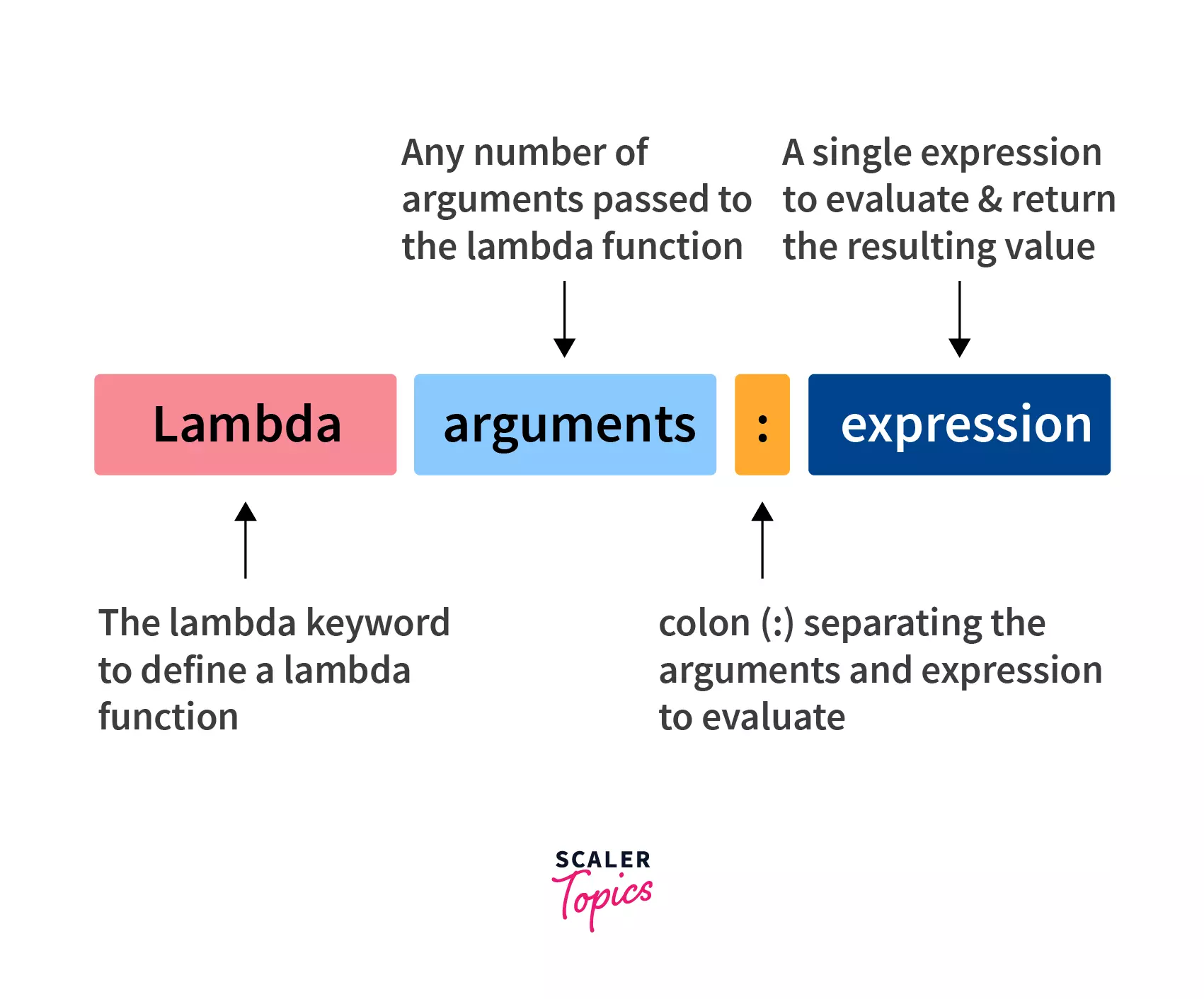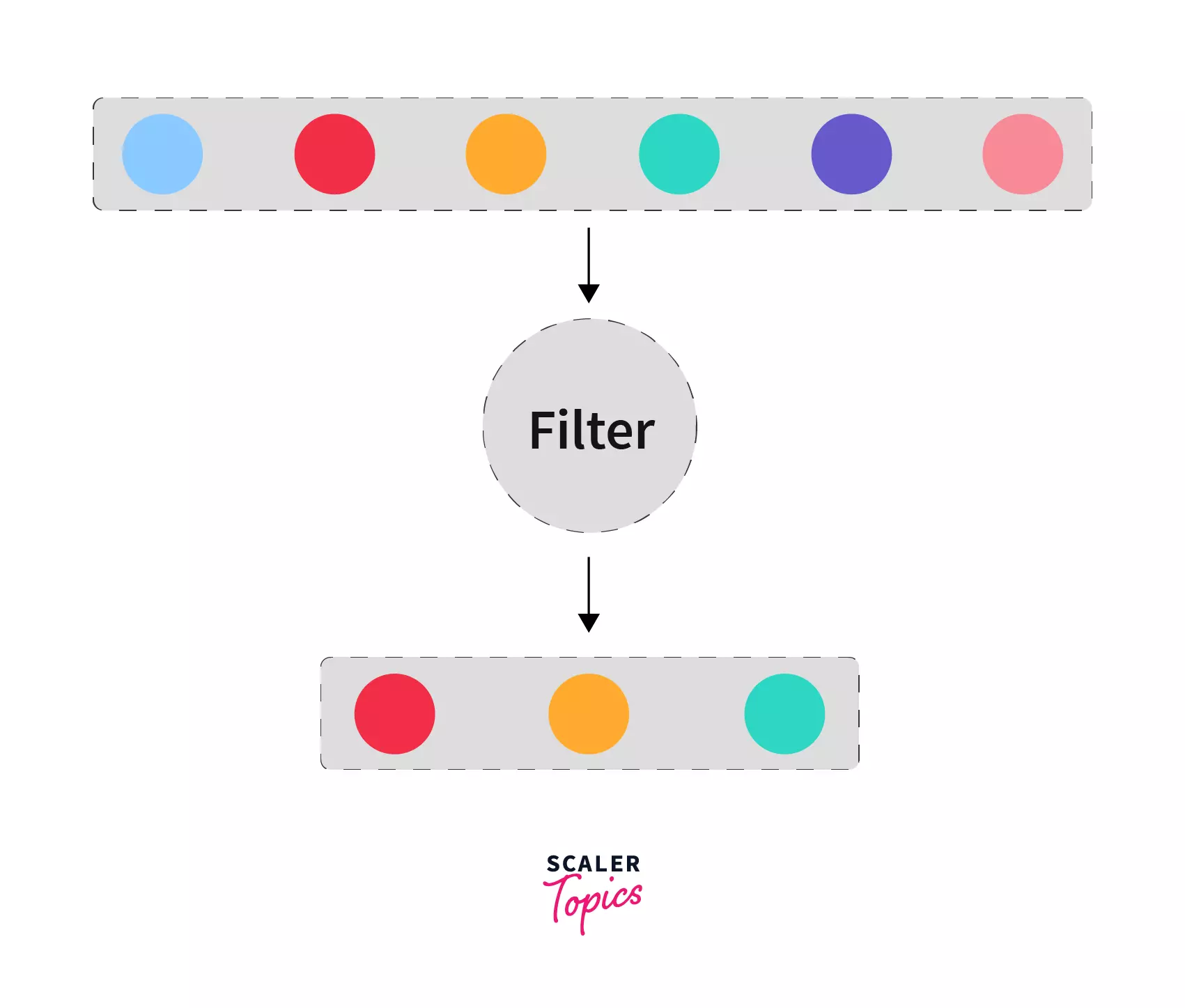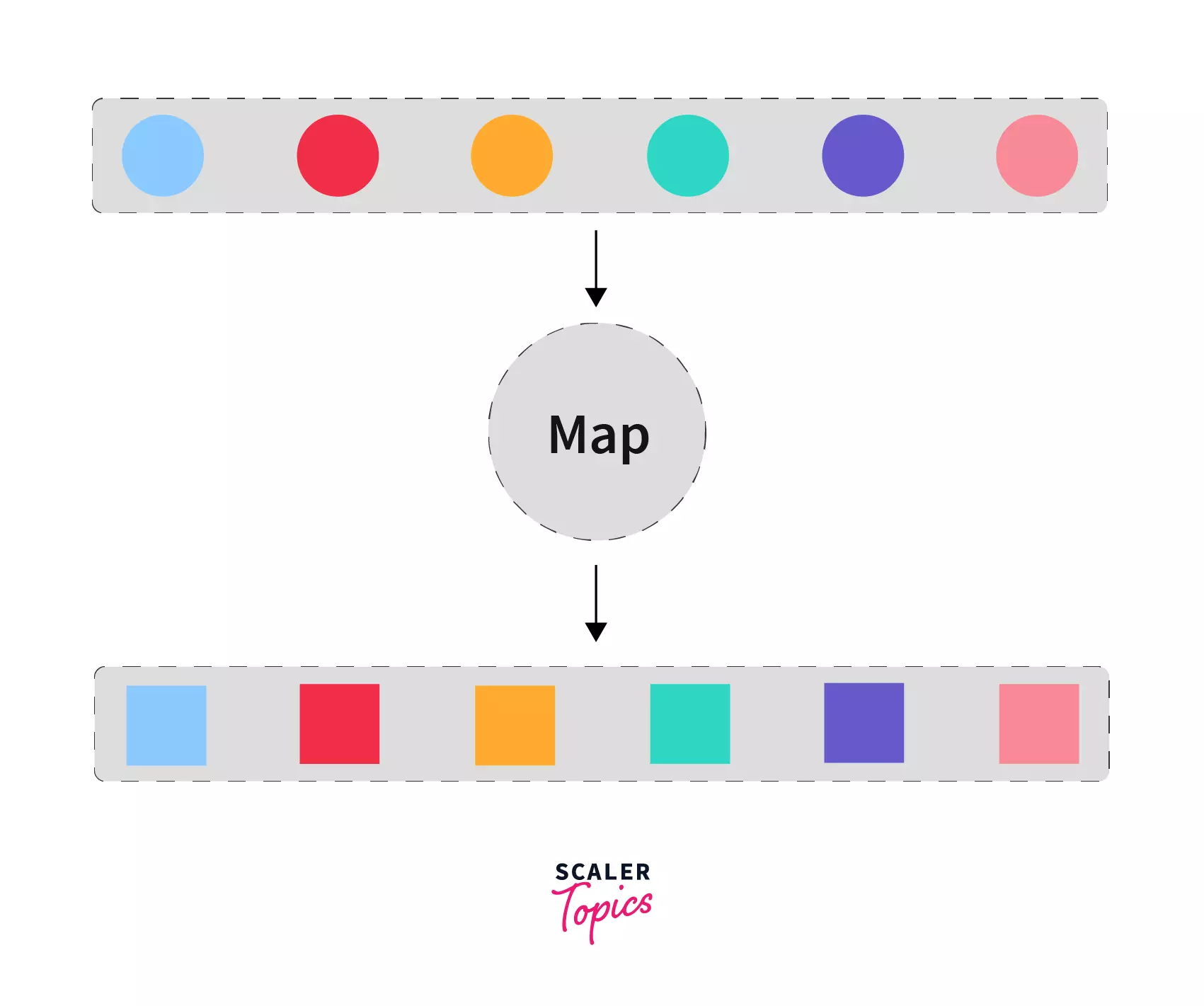Certificate

### Learn on Scaler Topics and get certified.# How to use Lambda Functions in Python?

Learn how to use lambda functions in python.

## Abstract

Lambda functions are functions with no name, they take any number of arguments and contain a single expression.
The expression is evaluated on the basis of arguments and the resulting value of the expression is returned automatically when the lambda function is invoked.
Lambda functions are mostly used with higher-order functions (functions that takes a function as an argument, or returns a function).

## Introduction to Python Lambda

Suppose we want to sort a list of names based on the last name. We can do this easily using the sort function of the list. The sort function provides a key parameter that accepts a function to serve as a basis for comparison:

``````names = ["Harry Potter", "Hermione Granger", "Ron Weasley", "Draco Malfoy"]

def get_last_name(fullname):
return fullname.split(" ")[-1].lower()

names.sort(key=get_last_name)

print(names)
``````

Output:

``````['Hermione Granger', 'Draco Malfoy', 'Harry Potter', 'Ron Weasley']
``````

Explanation: We created a get_last_name function that takes the full name as an argument and then returns the last name by:

• Splitting the name into different words using the space(" ") as a separator.
• Accessing the last name with [-1] indexing.
• And finally converting it into lowercase for case-insensitive comparison.

We used this function as a key to sort the names and then finally printed the sorted output.

Now, what if we only need the get_last_name function only once in our code?

For scenarios like this, Python provides a better approach using lambda functions.
In this article, we will learn what are lambda functions in python and how to use them to:

• Pass functions as an argument into another function
• Return a function from a function.

Takeaway

Lambda functions provide a way to write throwaway functions (functions that are needed for a short time).

## What are Lambda Functions in Python?

Python lambda functions are small, anonymous functions defined using the lambda keyword.

An anonymous function refers to a function declared with no name.

Let's see how you can define a python lambda function:

### Syntax of Python Lambda

``````lambda arguments: expression
``````

A lambda function can take any number of arguments including no argument, but they contain only one expression. The expression is evaluated and the resulting value is automatically returned by the lambda function.Takeaways:

• Lambda functions are small, anonymous functions defined using the lambda keyword.
• Lambda function can take any number of arguments but can return a single expression.

## Examples of Lambda Functions in Python

### Example 1: Sorting a list of names

At the beginning of this article, we discussed sorting a list of names based on the last name. Let's see how we can do that using a lambda function instead of a regular function defined with def.

``````names = ["Harry Potter", "Hermione Granger", "Ron Weasley", "Draco Malfoy"]

names.sort(key=lambda fullname: fullname.split(" ")[-1].lower())

print(names)
``````

Output:

``````['Hermione Granger', 'Draco Malfoy', 'Harry Potter', 'Ron Weasley']
``````

Explanation: We provided the following lambda function:

``````lambda fullname: fullname.split(" ")[-1].lower()
``````

as a key to the sorting function.

Our lambda function is taking one argument - fullname and then returning the last name by:

• Splitting the name into different words using the space(" ") as a separator.
• Accessing the last name with [-1] indexing.
• And finally converting it into lowercase for case-insensitive comparison.

Notice, that we don't need the return keyword inside a lambda function, the value is automatically returned.

### Example 2: Immediately Invoking Lambda Functions with No Arguments

As stated above, the lambda function can have any number of arguments including none:

``````(lambda : print("Hello, World!"))()
``````

Output:

``````Hello, World!
``````

Explanation: Here, we are defining a lambda function with no argument, therefore we wrote nothing between lambda and :. Then we are immediately invoking (calling) it using the (). The lambda function executes the print function resulting in Hello, World! as output.

### Example 3: Assigning Lambda Function to a Variable

We can reuse a lambda function by assigning it to a variable:

``````square = lambda x: x * x

print(square(2))
print(square(3))
``````

Output:

``````4
9
``````

Explanation: We created a simple lambda function that takes a number and squares it. Then we assigned that lambda function to an identifier named square. Now, square can be used like any regular function. We called it twice with 2 and 3. Thus getting their square - 4 and 9 in the output.

### Example 4: Python Lambda Function with Two Arguments

Let's write a lambda function that takes two numbers and subtract them:

``````subtract = lambda num1, num2: num1 - num2
result = subtract(6, 3)

print(result)
``````

Output:

``````3
``````

Explanation: Our lambda function takes two numbers: num1 and num2. We are subtracting num2 from num1 and returning the result which is finally printed on the screen.

Takeaways:

• We can use a lambda function as a key to the sort function of a list.
• A lambda function can be invoked immediately.
• We can assign a variable to a lambda function.

## Why Use Lambda Functions in Python?

Python lambda functions are used when we need a function to evaluate a simple expression for a short time.
The two most appropriate use cases of python lambda functions are with higher-order functions:

• Returning a function from a function.
• Passing a function to a function.

Higher-order function is a function that takes a function as an argument, or returns a function.

We should avoid writing lambda functions with complex expressions to maintain code readability.

For example:

``````(lambda x: x + (lambda a_list: sum(a_list))([1, 2, 3, 4, 5]))(100)
``````

Although the code is short, this expression contains a nested lambda expression hurting the readability and making it difficult to understand especially for beginners. We will later discuss more the pros and cons of using a lambda function.

Let's first discuss these two use cases in detail.

Takeaway:

• Lambda functions are mostly used with higher-order functions.

## Returning a Function from a Function

Consider the following function:

``````def make_multiplier_of(n):
return lambda x: n * x
``````

It is taking in a number n and returning a lambda function which accepts another number x and returns the multiplication of both the numbers that is n * x.

Now we can generate different functions using this one function:

``````times3 = make_multiplier_of(3)

times5 = make_multiplier_of(5)

print(times3(5))

print(times5(10))
``````

Output:

``````15
50
``````

Explanation: When we called the make_multiplier_of with n = 3. This value of n gets attached to the code inside of the nested lambda function which is then returned by the enclosing make_multiplier_of function. This technique by which some data gets attached to the code is called closure in Python. Now, when we call the times3 function, it is equivalent to calling the following function:

``````times3 = lambda x: 3 * x
``````

Takeaway:

• We can define closures using a lambda function.

## Passing a Function to a Function

Lambda functions are most commonly used when passing function objects as arguments to built-in functions like filter, map, sort, and even user-defined functions.

We have already seen the example of passing a key function to sort. Let's see some more examples with the filter and map functions.

### Use of Lambda Function in Python with filter()

The filter() function in Python takes in a function and a list as arguments. That function is called with all the items in the list and a new list is returned which contains items for which the function evaluates to True.The following example uses the filter function to filter out numbers greater than 10:

``````numbers = [4, 1, 7, 3, 6, 12, 18, 16, 50, 10, 4, 5]

numbers_gt_10 = list(filter(lambda x: x > 10, numbers))

print(numbers_gt_10)
``````

Output:

``````[12, 18, 16, 50]
``````

Explanation:

We created a list with some numbers, then used the filter function to filter out numbers that are greater than 10 by providing a lambda function that checks for the same: lambda x: x > 10. As the filter returns a filter object rather than a list, we have to convert its result to a list. Then, we finally printed the result.

### Use of Lambda Function in Python with map()

The map() function in Python also takes in a function and a list as arguments. That function is called for all the items in that list, and a new list is returned which contains items returned by that function.The following example uses the map function to double all the numbers in a list:

``````numbers = [1, 2, 3, 4, 5, 6]

doubles = list(map(lambda x: x * 2, numbers))

print(doubles)
``````

Output:

``````[2, 4, 6, 8, 10, 12]
``````

Explanation: We created a list with some numbers, then used the map function to double the numbers by providing the following lambda function: lambda x: x * 2. As the map returns a map object rather than a list, we have to convert its result to a list. Then, we finally printed the result.

Takeaway:

• Lambda functions are used to pass function objects to map and filter.

## Pros and Cons of Lambda Function in Python

Let's discuss some benefits of using lambda functions over regular functions:

### Pros

• Lambda functions make code shorter

With lambda functions, we can avoid defining functions that are only needed at a single place, thus making our code more concise.

• Useful in functional programming

As seen in the article, lambda functions are especially helpful when working with functions like sort and map (and many more...).

• Supports all the different ways of passing arguments We can pass any of the following argument to a lambda functions just like a regular function:
• Positional arguments Example: (lambda x, y: x + y)(1, 2)

• Keyword arguments Example: (lambda x, y: x + y)(1, y=2)

• Variable list of arguments Example: (lambda *args: sum(args))(1, 2)

• Variable list of keyword arguments Example: (lambda **kwargs: sum(kwargs.values()))(one=1, two=2)

• Keyword-only arguments

Takeaway:

• Lambda functions help to make code shorter and are often used in functional programming.

### Cons

To be honest, lambda functions should be used with caution. Lambda functions are often misused and lead to poor readability and non-pythonic code.

Let's go over some of the issues encountered when using lambda functions.

• No function name in Error Tracebacks

As lambda function has no name, if any Exception occurs in its execution, the traceback only shows <lambda>. Consider the following as an example:

``````
div_zero = lambda x: x / 0

print(div_zero(9))
``````

Output:

`````` Traceback (most recent call last):
File "divzero.py", line 3, in <module>
print(div_zero(9))
File "divzero.py", line 1, in <lambda>
div_zero = lambda x: x / 0
ZeroDivisionError: division by zero
``````

You can see the <lambda> in the fourth line of the output. This may cause trouble in debugging a program.

In contrast, if we have done the same thing with a regular function we would have got the function name as shown below:

`````` def div_zero(x):
return x / 0

print(div_zero(9))
``````

Output:

`````` Traceback (most recent call last):
File "divzero.py", line 4, in <module>
print(div_zero(9))
File "divzero.py", line 2, in div_zero
return x / 0
ZeroDivisionError: division by zero
``````

You can see the function name div_zero in the fourth line of the output.

• Lambda function can not contain any statements Statements like return, assert, pass, raise will raise a SyntaxError exception. Example:

``````(lambda x: assert x == 7)(7)
``````

Output:

``````File "<filename>", line 1
(lambda x: assert x == 7)(7)
^
SyntaxError: invalid syntax
``````
• Lambda functions can not have type annotations Type hints are a special syntax that allows declaring the type of a variable. You can use type hints in a regular function like this:

``````def add(x: int, y: int):
return x + y
``````

But doing so in a lambda function will raise a SyntaxError:

``````(lambda x: int, y: int: x + y)(1, 2)
``````

Output:

``````File "filename", line 1
(lambda x: int, y: int: x + y)(1, 2)
^
SyntaxError: invalid syntax
``````
• Lambda functions with complex expressions lead to poor readability Consider the following code as an example:

``````(lambda num: "One" if num == 1 else "Two" if num == 2 else "Three" if num == 3 else "Invalid number")(2)
``````

It is hard to understand what's going on here, let's re-write the above code as a regular function:

``````def one_two_three(num):
if num == 1:
return "One"
elif num == 2:
return "Two"
elif num == 3:
return "Three"
else:
return "Invalid number"

one_two_three(2)
``````

Now, it is quite evident that this function is returning "One", "Two", "Three" based on the number passed.

• Assigning a lambda function to a variable violates the PEP-8 style guide PEP-8 is a document that provides guidelines and best practices on how to write Python code.

PEP-8 states:

Always use a def statement instead of an assignment statement that binds a lambda expression directly to an identifier. (Source)

Assigning a lambda function to a variable means that we are reusing the function. Thus it will be better if we just define a regular function instead of a lambda function.

For example, if we are using the is_even function multiple times, we should define it as follows:

`````` def is_even(num):
return num % 2 == 0
```
```
is_even = lambda num: num % 2 == 0
``````
• Whenever possible we should use the more pythonic list comprehension over lambda functions

List comprehension is an elegant way to define and create lists based on existing lists.

• Using list comprehensions as an alternative to map Consider the following code shown before:

``````numbers = [1, 2, 3, 4, 5, 6]

doubles = list(map(lambda x: x * 2, numbers))

print(doubles)
``````

We can do the same thing using list comprehension as follow:

``````numbers = [1, 2, 3, 4, 5, 6]

doubles = [x * 2 for x in numbers]

print(doubles)
``````

This eliminated the need for defining and invoking a lambda function. Also, this is more expressive than a cryptic lambda function.

• Using list comprehensions as an alternative to filter Now, consider the following code shown before:

``````numbers = [4, 1, 7, 3, 6, 12, 18, 16, 50, 10, 4, 5]

numbers_gt_10 = list(filter(lambda x: x > 10, numbers))

print(numbers_gt_10)
``````

We can achieve the same result using list comprehension as follow:

``````numbers = [4, 1, 7, 3, 6, 12, 18, 16, 50, 10, 4, 5]

numbers_gt_10 = [x for x in numbers if x > 10]

print(numbers_gt_10)
``````

Again, this saves us from defining and invoking a lambda function and looks much cleaner.

Takeaway:

• Lambda functions can lead to several issues in the development when not used properly.

## Conclusion

• Lambda functions are small, anonymous functions defined using the lambda keyword.
• A lambda function can take any number of arguments, but they contain only one expression.
• Lambda function automatically return the evaluated value of the expression.
• Lambda functions are mostly used with higher-order functions.
• Lambda functions help to make code shorter but can lead to several issues in development like:
• Imprecise tracebacks.
• Inability to include statements like raise in the function body.
• No type annotation support.